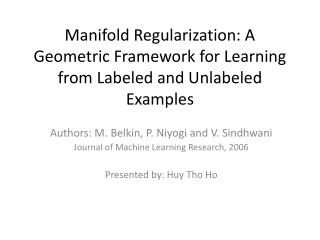# Manifold Regularization: A Geometric Framework for Learning from Labeled and Unlabeled Examples - PowerPoint PPT PresentationDownload PresentationManifold Regularization: A Geometric Framework for Learning from Labeled and Unlabeled Examples

Manifold Regularization: A Geometric Framework for Learning from Labeled and Unlabeled Examples
Download Presentation## Manifold Regularization: A Geometric Framework for Learning from Labeled and Unlabeled Examples

- - - - - - - - - - - - - - - - - - - - - - - - - - - E N D - - - - - - - - - - - - - - - - - - - - - - - - - - -
##### Presentation Transcript

1. Manifold Regularization: A Geometric Framework for Learning from Labeled and Unlabeled Examples Authors: M. Belkin, P. Niyogi and V. Sindhwani Journal of Machine Learning Research, 2006 Presented by: HuyTho Ho

2. Overview • Introduction • Reproducing Kernel Hilbert Space • Standard learning framework • Semi-supervised learning framework with geometric regularization • LaplacianRegularized Least Squares • Unsupervised and fully-supervised learning • Experiments

3. Introduction • 2 labeled examples • Prior notion of simplicity

4. Introduction • Additional unlabeled examples • Geometric structure of marginal distribution

5. Reproducing Kernel Hilbert Space • Hilbert space : • Real or complex inner product space • Complete metric space • Reproducing Kernel Hilbert Space (RKHS): • is an arbitrary set • is a Hilbert space of functions on • is a RKHS if every linear map of the form from to the complex numbers is continuous for

6. Standard Learning Framework • : a Mercer kernel • : associated RKHS of functions with norm • Standard framework • is the loss function: • : regularized least squares (RLS) • : support vector machines (SVM) • Classical Representer Theorem:

7. Geometric Regularization • New objective function: • reflects the intrinsic structure of • If is known, we have the new Representer Theorem: where • Both regularizers are needed: • True underlying marginal distribution is usually not known. • Manifold assumption may not hold.

8. Geometric Regularization • If is not known, is approximated by labeled and unlabeled data • Given : label data and : unlabeled data, the optimization problem becomes where : edge weights : graph Laplacian : diagonal matrix where

9. Geometric Regularization • Representer Theorem: • Remark: the normalized graph Laplacian performed better in practice

10. Regularized Least Squares • Objective function: • Representer Theorem: • Replace into the objective function: where is the Gram matrix, is the label vector • Solution:

11. Laplacian Regularized Least Squares • Objective function: • Representer Theorem: • Solution: where and

12. Unsupervised Learning • Objective function: • Approximation: • Using Representer Theorem

13. Fully-Supervised Learning • Objective function for a 2 class problem:

14. Experiments – Two Moon Dataset

15. Experiments – Hand Digit Recognition • USPS dataset • 45 binary classification problems

16. Conclusions • A framework for data-dependent geometric regularization • New Representer Theorem • Semi-supervised learning • Unsupervised learning • Fully-supervised learning • Pros: • Exploit the geometric structure of the marginal distribution of training samples. • Cons: • The marginal distribution does not have any geometric structure. • The geometric structure of the marginal distribution is hard to recover.